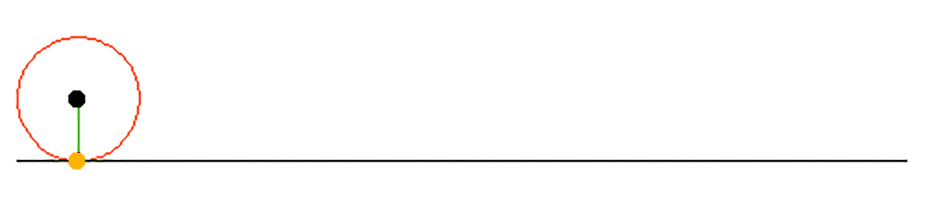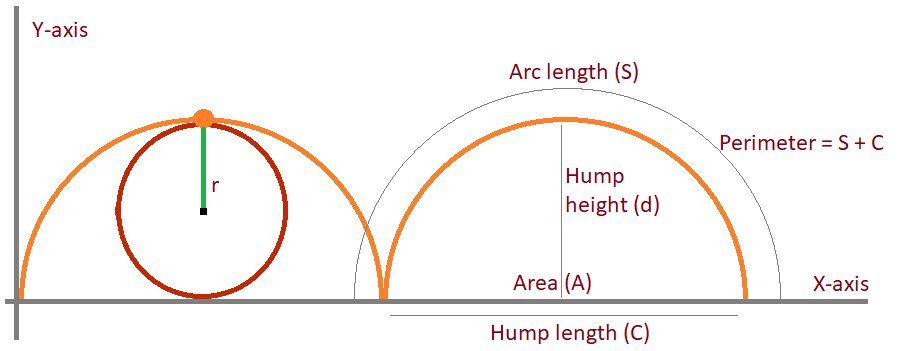# Cycloid Calculator

Created by Komal Rafay
Reviewed by Hanna Pamuła, PhD candidate and Jack Bowater
Last updated: Feb 15, 2022

The cycloid calculator allows you to calculate the necessary parameters required to generate a cycloid. Our tool mainly requires the radius of the circle, from which it can find the area, arc length, hump length, height, and the perimeter of each arc of the cycloid.
We are sure you would like to know how to construct a cycloid or how cycloid curve tracing is done? We will go through all these topics, so continue reading below.

## What is a cycloid?

Try to picture a dropped coin rolling along a straight line. Now imagine a point anywhere on the edge of that coin. That point is tracing a curve as it moves. Were you able to picture that? Because that is a cycloid. In other words or more technical words, a cycloid is a curve formed by a circle rotating along a straight line.
The history of the cycloid is a controversial one, as many mathematicians have claimed to have discovered it. But Galileo was the first one to study the curve in a methodical way and gave it the name cycloid.## Cycloid equation

A cycloid comprises various components, the most important one being the radius of the rolling circle that forms the cycloid. All the components of a cycloid along with their equations are given below:

• Polar equation of cycloid

The polar equations of cycloid are given as:
x = r*(θ - sin(θ))
y = r*(1 - cos(θ))

Where,

• θ - Angular displacement of the circle.
• Cartesian equation

The Cartesian equation of cycloid is given as:
 x = r* cos ⁻1 (1 - y/r ) - √ y*(2 r - y)

The arc length of a cycloid is the curved distance between two cusps. It is denoted by S and its formula is:
S = 8*r

• Area of a cycloid

The area of a cycloid is the area under the arch, which is created by a single revolution of the circle. As used in the cycloid calculator, its formula is given by:
A = 3*π*r²

• Hump length of a cycloid

The hump length is the straight line distance between two cusps. It is also the circumference of the rolling circle, and its formula is:
C = 2*π*r

• Hump height of a cycloid

The hump height of a cycloid is the height of the arch, and it is equivalent to the diameter of the rotating circle. Its formula is:
d = 2*r

• Perimeter of a cycloid

The perimeter of a cycloid is the sum of the lengths of all its sides. The cycloid comprises 2 sides, the arch and the base. To obtain the perimeter, we need the hump and arc lengths. Its formula is:
p = C+S

## How to construct a cycloid

Now that you have used our cycloid calculator, you know what parameters are used for cycloid curve tracing.
But some of you will be wondering how you would construct a cycloid on paper if needed. Follow the steps given below to construct your own cycloid:

Take the circle diameter as 5 cm or 50 mm.

1. Draw a circle of radius of 2.5 cm or 25 mm and mark its center as C.

2. Now, draw a horizontal line the same length as the circle's circumference from the base and center, C.

3. Now divide the circle, horizontal line, and the base-line into 12 equal parts. Name the points on the horizontal line as C1, C2, C3 till C12 and 1,2,3, till 12 on the base-line.

4. Join C1 with 1, C2 with 2, C3 three with 3, and continue doing so till C12 to 12.

5. Draw horizontal lines from all the points on the circle in the same direction as the horizontal line drawn from the center before.

6. Taking center C1 and radius 2.5 cm or 25 mm, draw an arc on the line which passes through 1. Then taking the center C2 and radius 2.5 cm or 25mm, draw an arc passing through 2. Similarly, taking center C3 and radius 2.5 cm or 25mm, draw an arc on the line passing through 3. Keep following the same procedure for all points on the main horizontal line till C12.

7. Using the arcs you made, draw a freehand smooth curve.This is the curve traced by a point on the circle's circumference if it roll along a straight line without slipping, also known as your cycloid.

## Cycloid curve

A cycloid curve follows simple harmonic motions, which means it is periodic and will replicate an identical pattern for each revolution.
The concept of the cycloid curve or cycloidal arch has been used in the architectural design of the Kimbel Art Museum in Texas.

## Variations of a cycloid

There are several variations of a cycloid. They usually differ in the point on the rolling circle that creates the curve. In some cases, the point can be inside or outside the circle, which gives curtate or prolate cycloid, respectively.
A list of variations of a cycloid is given below:

• Curtate cycloid;
• Prolate cycloid;
• Trochoid;
• Hypocycloid;
• Epicycloid;
• Hypotrochoid; and
• Epitrochoid.

## FAQ

### How to find the arc length of a cycloid?

The formula to find out the arc length of a cycloid is given as:
S = 8 × r
Where S is arc length, and r is the radius of the circle.

### What is the formula of area of cycloid?

The area of a cycloid is the area under the arch of a cycloid. Its formula is:

A = 3 × π × r²

### What does the cycloid calculator calculate?

The cycloid calculator takes the radius of the rolling circle as input and calculates the following components of a cycloid:

• Arc length;
• Area;
• Hump length;
• Hump height; and
• Perimeter.
Komal Rafaym
Area of cycloid (A)
Arc Length (S)
m
Hump Height (d)
m
Hump Length (C)
m
Perimeter of cycloid
m
People also viewed…

### Circumference

Use this free circumference calculator to find the area, circumference and diameter of a circle.

### Cylindrical coordinates

Cylindrical coordinates calculator converts between Cartesian and cylindrical coordinates in a 3D space.

### Partial products

The partial products calculator can show you how to calculate the product of any two numbers using the well-beloved partial products algorithm.